Courses

# Test: Division Algorithm For Polynomials

## 20 Questions MCQ Test Mathematics (Maths) Class 10 | Test: Division Algorithm For Polynomials

Description
This mock test of Test: Division Algorithm For Polynomials for Class 10 helps you for every Class 10 entrance exam. This contains 20 Multiple Choice Questions for Class 10 Test: Division Algorithm For Polynomials (mcq) to study with solutions a complete question bank. The solved questions answers in this Test: Division Algorithm For Polynomials quiz give you a good mix of easy questions and tough questions. Class 10 students definitely take this Test: Division Algorithm For Polynomials exercise for a better result in the exam. You can find other Test: Division Algorithm For Polynomials extra questions, long questions & short questions for Class 10 on EduRev as well by searching above.
QUESTION: 1

### The expression that should be subtracted from the polynomial f(x) = x4 + 2x3-13x2 – 12x + 21 so that the resulting polynomial is exactly divisible by g(x) = x2 – 4x + 3 is​

Solution:

Step-by-step explanation:

Given: Dividend =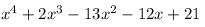Divisor =  x2 - 4x + 3

Solution :

Since we know that :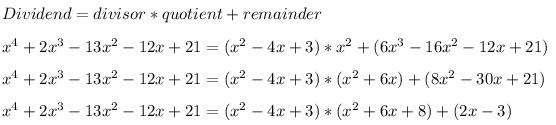Since the remainder is  2x-3

So, 2x-3 must be subtracted from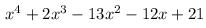so that the resulting  polynomial is exactly divisible by x2 - 4x + 3

QUESTION: 2

### If (x + 1) is a factor of x2 – 3ax + 3a – 7, then the value of a is

Solution:

Since (X+1) is a factor of x2 - 3ax + 3a - 7

Therefore , x+1=0

or, x=-1

Putting the value of x in the the p(x) = x2 - 3ax + 3a - 7

or, (-1)2 -3(a)(-1)+3a-7=0

1+3a+3a-7=0

6a=6

or, a=1

Therefore the  value of a=1.

QUESTION: 3

### The value of quadratic polynomial f (x) = 2x2– 3x- 2 at x = -2 is ……​

Solution:

We put the value x=-2 in the polynomial 2x2-3x-2
f(-2)=2(-2)2-3(-2)-2=2*4+6-2=12

QUESTION: 4

If the degree of the dividend is 5 and the degree of the divisor is 3, then the degree of the quotient will be

Solution:

The degree of the divisor is 2

Step-by-step explanation:

x5÷x3=x2

QUESTION: 5

The value of 155 mod 9 is

Solution:

By the Division algorithm 155 = 9(17) + 2. Where remainder is 155 mod 9.

QUESTION: 6

On dividing f(x) = x3 – 3x2 + x + 2 by a polynomial g(x) the quotient and remainder q(x) and r(x) are (x – 2) and (-2x + 4) respectively, then g(x) is​

Solution:

Divisor*Quotient+Remainder= Divident
g(x)(x-2)+(-2x+4)=x3-3x2+x+2
g(x)(x-2)=x3-3x2+x+2+2x-4
g(x)(x-2)=x3-3x2+3x-2
g(x)=x3-3x2+3x-2/(x-2)
g(x)=x2-x+1

QUESTION: 7

The three zeroes of the polynomial 2x3 + 5x2 – 28x – 15 _____

Solution:

Since the solution is not a complex number, it is a real number. Since rational numbers include all integers, natural numbers and fractions , So the numbers are rational numbers.

QUESTION: 8

If two of the zeroes of the polynomial f (x) = x4 – 3x3 – x2 + 9x – 6 are -√3 and √3 then all the zeroes are​

Solution:
QUESTION: 9

If (x + 1) is a factor of 2x3 + ax2 + 2bx + 1, then find the values of a and b given that 2a – 3b = 4.​

Solution:

Given is the value of a and b.

given that (x+1) is a factor of p(x)

therefore, -1 is a zero of given p(x)

p(x) = 2x3 +ax2 +2bx +1

substituting the value of -1 in the given p(x), we get

p(x)=2*(-1)3 +a *(-1)2 +2*b*(-1)+ 1

= -2 + a -2b + 1

= -1 + a - 2b

or,a - 2b = 1

also given that 2a - 3b = 4

so we got two equations;

a - 2b = 7 ...(1)

2a - 3b = 4 ...(2)
(1)* (2)* (4)- 2 = 2a - 4b = (3)

(2)* 1 = 2a - 3b = 4 (4)

(4) -  (3) = [2a - 2a ] + [-3b - (-4b)] = 4 - 2

-3b + 4b = 2

therefore b = 2

substituting the value of bin (3)

2a - 4b = 2

2a - (4*2) = 2

2a - 8 = 2

2a = 2 + 8

2 = 10

a = 10 / 2

therefore a = 5

so we get the value of a and b

that is ; a = 5 and b = 2

QUESTION: 10

Which of the given is the set of zeroes of the polynomial p(x) = 2x3 + x2 – 5x + 2

Solution:

We have the polynomial 2x3+x2-5x+2
By Hit and trial method, x=1 is the solution.
Dividing the polynomial by x-1
We get quotient as = 2x2+3x-2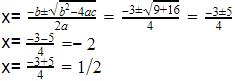QUESTION: 11

The value of p when x3 + 9x2 + px – 10 is exactly divisible by (x+ 2) is ____

Solution:
QUESTION: 12

When the polynomial f(x) = 4x3 + 8x2 + 8x + 7 is divided by the polynomial g(x) = 2x2 – x + 1, the quotient and the remainder are​

Solution:
QUESTION: 13

When x2 – 2x + k divides the polynomial x4 – 6x3 + 16x2 – 25x + 10, the remainder is (x + a). The value of a is _________

Solution:

Given that the remainder is (x + a)
⇒ (4k – 25 + 16 – 2k)x + [10 – k(8 – k) ] = x + a
⇒ (2k – 9)x + [10 – 8k + k2 ] = x + a
On comparing both the sides, we get
2k  – 9 = 1
⇒ 2k = 10
∴ k = 5
Also 10 – 8k + k2 = a
⇒ 10 – 8(5) + 52 = a
⇒ 10 – 40 + 25 = a
∴ a = – 5

QUESTION: 14

If the degree of the divisor g(x) is one then the degree of the remainder r(x) is

Solution:

Degree of remainder is one less than the degree of the divisor because if the remainder is of the same degree then it can be divided further , its just like when the divisor is a number the remainder is less than the number because if it is more than or equal to that number it can further be divided. So, the degree is zero.

QUESTION: 15

If the polynomial (2x + 3) is a factor of the polynomial 2x3 + 9x2 – x – b. The value of b is_______

Solution:

let the given polynomial be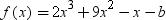since 2x+3=0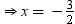if (2x+3) is a factor of f(x). therefore by the factor theorem f(-3/2) =0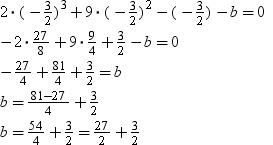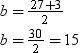QUESTION: 16

If two of the zeros of the polynomial x4 – 17x3 + 90x2 – 166x + 92 are (7 + √3) and (7 – √3), then the other two zeros are _________​

Solution:
QUESTION: 17

If f(x) is divided by g(x), g(x) ≠ 0, then there exist two polynomials q(x) and r(x) such that​

Solution:

Dividend = Divisor × Quotient + Remainder.
When we divide f(x) by g(x) then q(x) is quotient and r(x) is remainder.
Therefore, f(x) = g(x) × q(x) + r(x).

QUESTION: 18

The expression that should be added to the polynomial f(x) = x4 + 2x3 – 2x2 + x + 1, so that it should be exactly divisible by (x2 + 2x – 3) is​

Solution:
QUESTION: 19

When x3 – 3x2 + 5x – 3 is divided by x2 – k , the remainder is 7x + a . Then the value of k is_____

Solution:
QUESTION: 20

If two zeroes of a polynomial 4x4 -20x3 +23 x2 + 5x – 6 are ½ and – ½, the how many more zeroes does it have?

Solution:

The polynomial with degree 4 is a biquadratic equation. And a polynomial has no. of zeros equal to the degree of the polynomial. So total no. of zeros are 4. 2 zeros are already provided to us , so it has 2 more zeros.